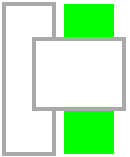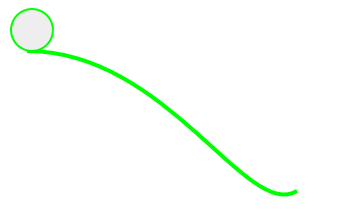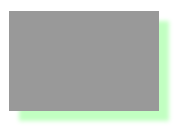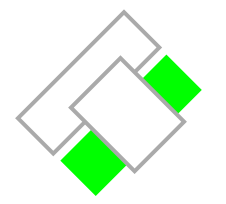There is a great tendency among web browsers nowadays - the wide and fast adoption of HTML5 APIs. Why should we, as web developers, be excited about it? Well let's take the canvas tag, for example. Imagine that you can render raster graphics in the browser, edit an image purely on the client-side, apply filters on it or draw complex animations without the need for plugins, just JavaScript. Imagine the power and features you can implement without bothering with the overhead and complexity of server-side calls. This all and much more is possible with the canvas tag. In this blog post I will try to tip the top of the iceberg just to give you a taste of the mastering the client-side raster graphics.

## Getting to know <canvas>

Let’s unveil the mysteries of the canvas tag. Introduced in 2004 by Apple in their WebKit implementation, the element has gained wide acceptance among the web community and inevitably has found its place as part of the HTML5 final draft specification (or at least the 2D context did). The canvas tag, as part of the HTML5 specification, provides a media for rendering raster graphics in the browser using only JavaScript. In order to operate in 2D space (or any space for that matter) a context needs to be created, through which the graphics API can be accessed and used for drawing geometries and per-pixel editing.

Its distinctive quality is that it is a low-level, procedural model. This means that rendering happens on the fly through method calls. The context object that operates on the canvas stores particular state/settings that define the drawing patterns.

## The fun part

Having completed the abstract view on the canvas tag through this short theoretical introduction it is time to get our hands dirty.

### Getting the context

To start we need a simple HTML page with a defined canvas element:

`<canvas id=``"canvas2d"` `width=``"600"` `height=``"400"``> `
`    ``This message appears ``if` `canvas is not supported. `
`</canvas>`

The first thing to do is get the drawing context that executes the drawing methods on the canvas. In order to do this, simply use the getContext method of the canvas element providing the name of the respective implementation – 2d, 3d, WebGL, etc. Check http://www.webgl.com/ for more information on an implementation of a 3D context based on OpenGL.

`function` `getCanvas2dContext(canvasId) { `
`    ``var` `canvas = document.getElementById(canvasId); `
`    ``if` `(canvas && canvas.getContext) `
`        ``return` `canvas.getContext(``"2d"``); `
`}`

### Let’s draw… rectangles

Once we have the context it’s time to do the first drawing. We will start by drawing rectangles. There are two methods for that – strokeRect and fillRect that both accept 4 parameters – startX, startY, endX, endY. The positions specified here (and in any canvas drawing method) are in pixels and are relative to the top-left corner of the canvas element.

`function` `drawRectangles(context) { `
`    ``context.fillStyle = ``'#0f0'``; ``//green `
`    ``context.strokeStyle = ``'#aaa'``; ``//grey `
`    ``context.lineWidth = 4; `
` `
`    ``context.strokeRect(0, 0, 50, 150);``//draws only the sides of the rectangle `
`    ``context.fillRect(60, 0, 50, 150);``//fills the whole rectangle `
`    ``context.clearRect(30, 35, 90, 70);``//clears any drawing in the defined rectangle `
`    ``context.strokeRect(30, 35, 90, 70); `
`}`

Check the way the third rectangle appears to be drawn over the others, thanks to the clearRect method call.### Paths and curves

The 2d context of the canvas tag offers great capabilities for drawing any form of paths – will it be straight lines or curves of any kind. The drawing should be defined between beginPath and closePath calls. For straight lines one can use the moveTo(x,y) and lineTo(x,y) methods. When using these two methods the drawing is done in the following fashion – moveTo lifts the brush and positions it at the specified location, whereas lineTo uses the brush to draw a line to the specified position:

`function` `drawPath(context) { `
`    ``//set some styling properties. `
`    ``context.fillStyle = ``'#eee'``; `
`    ``context.strokeStyle = ``'#0f0'``; `
`    ``context.lineWidth = 4; `
` `
`    ``context.beginPath(); ``//starts the sequence of path drawing `
`    ``context.moveTo(10, 100); ``// this is the start point `
`    ``context.lineTo(130, 100); `
`    ``context.lineTo(70, 10); `
`    ``context.lineTo(10, 100); `
`    ``context.lineTo(130, 100);``//just to complete the rectangle edge `
` `
`    ``//NB: the geometry is not visible until stroke and/or fill method are called `
`    ``context.fill(); `
`    ``context.stroke(); `
`    ``context.closePath(); `
`}`

The code above will produce an equilateral triangle, where the side will be 130px-10px=120px.

There are different methods for drawing curves, but for the sake of brevity, we will focus on bezierCurveTo (drawing Bézier Curves) and arc (drawing circles or sectors):

`function` `drawCurves(context) { `
`    ``context.fillStyle = ``'#eee'``; `
`    ``context.strokeStyle = ``'#0f0'``; `
`    ``context.lineWidth = 4; `
` `
`    ``//draw the slope using a Bèzier curve `
`    ``context.beginPath(); `
`    ``context.moveTo(30, 50); `
` `
`    ``//arguments: cp1x, cp1y, cp2x, cp2y, x, y /* where cp => control point */ `
`    ``context.bezierCurveTo(170, 50, 250, 220, 300, 190); `
`    ``context.stroke(); `
`    ``context.closePath(); `
` `
`    ``//draw the ball using the arc method `
`    ``context.beginPath(); `
` `
`    ``//arguments: x, y, radius, start angle, end angle, anticlockwise `
`    ``context.arc(35, 29, 20, 0, 360, ``false``); `
`    ``context.stroke(); `
`    ``context.fill(); `
`    ``context.closePath(); `
`}`

This code snippet will produce the following raster:In there you can see the Bézier Curve as the slope. The circle above it is the result of the arc method call closing the path at 360deg.

### Draw Text

Being able to draw text is essential and very useful. In the 2d context of the canvas there are the two methods to do this – fillText(text, x, y) that draws a solid text and strokeText(text, x, y) that draws the borders of the letters without filling them. Of course, the canvas provides settings to change the font and text position. Here is a short example:

`function` `drawText(context) { `
`    ``context.fillStyle = ``'#00f'``; `
`    ``context.font  = ``'italic 30px sans-serif'``; `
`    ``context.textBaseline = ``'top'``; `
`    ``context.fillText (``'Hello world!'``, 0, 0); `
`    ``context.font = ``'bold 30px sans-serif'``; `
`    ``context.strokeText(``'Hello world!'``, 0, 50); `
`}`

Drawing shadows is part of the API as well. Almost all basic characteristics of shadows are covered by the canvas context – the shadow offset, color, blur (Gaussian Blur):

`function` `drawShadows(context) { `
`    ``context.shadowOffsetX = 10; `
`    ``context.shadowOffsetY = 10; `
`    ``context.shadowBlur = 4; `
`    ``context.shadowColor = ``"rgba(100, 255, 100, 0.4)"``; `
`    ``context.fillStyle = ``"#999"``; `
`    ``context.fillRect(20, 20, 150, 100); `
`} `

The code above will result in the following canvas rendering, where the green neon shadow is clearly visible.### Per-pixel editing

Along with the ability to draw geometries through the graphics API, the context offers a way to manipulate each pixel individually. The canvas is represented by a one dimensional array of values (vector) between 0-255, where each pixel is represented by a 4-tuple of neighboring elements in the combination RGBA(red, green, blue, alpha). This array can be accessed through the getImageData method and after the manipulations put back in the canvas through setImageData.

Here is an example that creates the negative of the rendered image. This is done by subtracting the color components of the pixel from 255, thus leaving only the negative color value for the pixel.

`function` `invertColorFilter(context) { `
`    ``//store the dimensions of the canvas `
`    ``var` `size = {width: context.canvas.width, height: context.canvas.height}; `
`                 `
`    ``//get the CanvasPixelArray from the given coordinates and dimensions. `
`    ``var` `imgd = context.getImageData(0, 0, size.width, size.height); `
`    ``var` `pix = imgd.data; `
` `
`    ``//iterating over 4 in order to go to the next pixel 4-tuple. `
`    ``for` `(``var` `i = 0, n = pix.length; i < n; i += 4) { `
`        ``pix[i  ] = 255 - pix[i  ]; ``//red `
`        ``pix[i+1] = 255 - pix[i+1]; ``//green `
`        ``pix[i+2] = 255 - pix[i+2]; ``//blue `
`    ``} `
` `
`    ``//put back the changed image data `
`    ``context.putImageData(imgd, 0, 0); `
`}`

### Transformations and animating the canvas

It is common for any graphics API to support linear transformations and the 2D canvas context is no different. Along with short-hand functions such as translate, rotate, scale, etc. that cover the basic linear transformations, there are the more complex methods transform(m00,m10, m01, m11, m02, m12) and setTransform(m00,m10, m01, m11, m02, m12). The last two methods provide directly a matrix to the transformation engine. The transform method just adds another transformation in the queue, while setTransform defines the new transformation matrix, thus resetting the previously defined transformations. The transformation matrix(3x3) definition is the following:

 m00 m01 m02 m10 m11 m12 0 0 1

In that matrix m02 and m12 define the translation of the objects, whereas the m00 – m11 define the linear transformation itself in 2D space. To be more precise this matrix describes affine transformations – linear transformations followed by a translation.

Here is a simple example of rotating the rectangles from the first example:

`function` `drawRotate(context) { `
`    ``context.translate(200, 100); `
`    ``context.rotate(deg2rad(45)); `
`    ``drawRectangles(context); `
`}`

Results in:The translation is needed so that no part of the rectangles in clipped on the canvas edges.

Being able to transform objects in the canvas, putting this to animation seems like the next natural step. In order to make it work, we need to call an animation handler repeatedly over a specific period of time. Since the introduction of the requestAnimationFrame, setting up the animation has become even easier. Here is a simple example of making the rectangles rotate:

`function` `animateBoxes(context) { `
`    ``var` `angle = 0; `
`    ``var` `animation = ``function``() { `
`        ``angle = ++angle % 360; ``//calculate the new angle of rotation `
`        ``context.save(); ``//save the context so that other transformations do not interfere `
`        ``context.clearRect(0, 0, context.canvas.width, context.canvas.height); ``//clear the canvas, otherwise artifacts from the previous animation frames will be visible `
`                     `
`        ``context.translate(150, 150); `
`        ``context.rotate(deg2rad(angle)); `
`        ``context.translate(-50, -50); `
`                     `
`        ``drawRectangles(context); `
`        ``context.restore(); `
`    ``}; `
` `
`    ``if``(``typeof``(requestAnimationFrame) != ``"undefined"``) `
`        ``requestAnimationFrame(animation); `
`    ``else`
`        ``setInterval(animation, 15); `
`}`

One important aspect of doing transformation in canvas is the way they are stacked. The most recent definitions are applied first, which resembles the behavior of a LIFO queue. When building animations one should keep this in mind.

The provided examples and sample code are combined in a sample web site. It is written in pure HTML, CSS and JavaScript with few helper methods from jQuery. Therefore they are easy to check out and run. So give it a try.

When we were first building the Telerik’s ASP.NET Image Editor control we had the idea that sooner or later we will integrate the canvas tag support in it and that day is coming pretty close. Being impressed by the productivity and capabilities of the canvas tag in modern browsers and being able to edit the image without the unnecessary going back and forth to the server we hope that the ImageEditor will become ever better at what it does. Furthermore it will provide more features for the near future when we will most likely implement various filters and drawing tools.

I hope this small introduction to the marvelous world of the 2D context of the canvas tag has been useful and fun for you. Your feedback on the content or features we can implement in RadImageEditor are more than welcome.